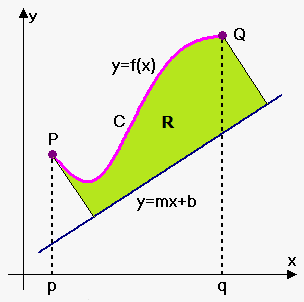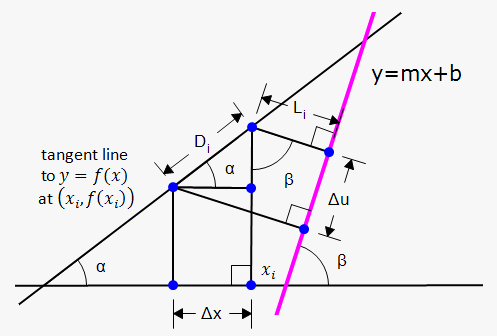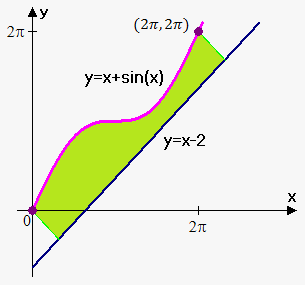# Volume of Solid of Revolution about Slant Line

We know how to find the volume of a solid of revolution obtained by rotating a region about a horizontal or vertical line (see Method of Disks/Rings and Method of Cylindrical Shells). But what if we rotate about a slanted line, that is, a line that is neither horizontal nor vertical?Let C be the arc of the curve y=f(x) between the points P=(p,f(p)) and B=(q,f(q)) and let R be the region bounded by C , by the line y=mx+b (which lies entirely below C), and by the perpendiculars to the line from A and B.

We first will try to find area of region R. For this we will need another sketch.

We again approximate area by the sum or rectangles, but this time rectangles are perpendicular to the line y=mx+b. Area of i-th rectangle is L_iDelta u. Thus, approximate area of R is A~~sum_(i=1)^nL_i Delta u.

Taking n very large we have that A=lim_(n->oo)sum_(i=1)^n L_i Delta u.

We need to express L_i and Delta u in terms of Delta x.From the sketch we immediately have that tan(alpha)=f'(x_i) (slope of tangent line) and tan(beta)=m.

Also, D_i=(Delta x)/cos(alpha) , and Delta u=D_icos(beta-alpha)=D_i(cos(beta)cos(alpha)+sin(alpha)sin(beta)).

From this we have that Delta u=(Delta x)/(cos(alpha))(cos(beta)cos(alpha)+sin(alpha)sin(beta))=Delta x (cos(beta)+tan(alpha)sin(beta)).

Now, we need to express sin(beta) and cos(beta) in terms of tan(beta).

From identity tan^2(beta)+1=1/(cos^2(beta)) we have that cos(beta)=sqrt(1/(1+tan^2(beta)))=sqrt(1/(1+m^2)).

Since sin^2(beta)+cos^2(beta)=1 then sin(beta)=m/sqrt(m^2+1).

Therefore, Delta u=Delta x(1/(sqrt(m^2+1))+f'(x_i) m/sqrt(m^2+1))=Delta x (1+mf'(x_i))/(sqrt(m^2+1)).

Now, we need to determine L_i.

It is fairly easy to do: L_i=(f(x_i)-(mx_i+b))cos(beta)=(f(x_i)-mx_i-b)/(sqrt(m^2+1)).

That's all. A=lim_(n->oo)sum_(i=1)^n L_i Delta u=(f(x_i)-mx_i-b)/sqrt(m^2+1)*(1+mf'(x_i))/(sqrt(m^2+1))Delta x=1/(m^2+1)lim_(n->oo)sum_(i=1)^n(f(x_i)-mx_i-b)(1+mf'(x_i)).

This is limit of the Riemann sum, i.e. definite integral, so

Area of region R is A=1/(m^2+1)int_p^q (f(x)-mx-b)(1+mf'(x))dx.

Now, to find volume we use method of disks: we slice perpendicular to the line y=mx+b: radius of disk is L=(f(x)-mx-b)/sqrt(m^2+1).

Thus, A(x)=piL^2=pi((f(x)-mx-b)/(sqrt(m^2+1)))^2.

Therefore, V=int_p^q piL^2du=int_p^q pi((f(x)-mx-b)/sqrt(m^2+1))^2(1+mf'(x))/sqrt(m^2+1)dx.

Volume of Solid of Revolution around Slant Line y=mx+b is V=pi/(m^2+1)^(3/2)int_p^q(f(x)-mx-b)^2(1+mf'(x))dx.

Example. Find the area of region R bounded by f(x)=x+sin(x), by the line y=x-2, and by the perpendiculars to the line from P=(0,0) and Q=(2pi,2pi). Find volume of the solid obtained by rotating the region R about the line y=x-2.Here we have m=1 , b=-2 , f(x)=x+sin(x), thus, f'(x)=1+cos(x).

Therefore, A=1/(1^2+1)int_0^(2pi)(x+sin(x)-x+2)(1+1*(1+cos(x)))dx=

=1/2int_0^(2pi)(2+sin(x))(2+cos(x))dx=

=1/2 int_0^(2pi)(4+2cos(x)+2sin(x)+sin(x)cos(x))dx=

=1/2int_0^(2pi)(4+2cos(x)+2sin(x)+1/2sin(2x))dx=

=1/2(4x+2sin(x)-2cos(x)-1/4cos(2x))|_0^(2pi)=1/2(8pi-2-1/4+2+1/4)=4pi.

And volume is

V=pi/(1^2+1)^(3/2)int_0^(2pi)(x+sin(x)-x+2)^2(1+1*(1+cos(x)))dx=pi/sqrt(8)int_0^(2pi)(2+sin(x))^2(2+cos(x))dx=

=pi/sqrt(8)int_0^(2pi)(4+4sin(x)+sin^2(x))(2+cos(x))dx=

=pi/sqrt(8)int_0^(2pi)(8+4cos(x)+8sin(x)+4sin(x)cos(x)+2sin^2(x)+sin^2(x)cos(x))dx

Now we use double angle formulas and integral can be rewritten as

=pi/sqrt(8)int_0^(2pi)(8+4cos(x)+8sin(x)+2sin(2x)+1-cos(2x)+sin^2(x)cos(x))dx=

=pi/sqrt(8)int_0^(2pi)(9+4cos(x)+8sin(x)+2sin(2x)-cos(2x)+sin^2(x)cos(x))dx=

=pi/sqrt(8)(9x+4sin(x)-8cos(x)-cos(2x)-1/2sin(2x)+1/3sin^3(x))|_0^(2pi)=

=pi/sqrt(8)(18pi-8-1+8+1)=pi/sqrt(8)*18pi=(9pi^2)/sqrt(2) .

Note, how we used substitution u=sin(x) for integral int sin^2(x)cos(x)dx to obtain that int sin^2(x)cos(x)dx=1/3 sin^3(x)dx.